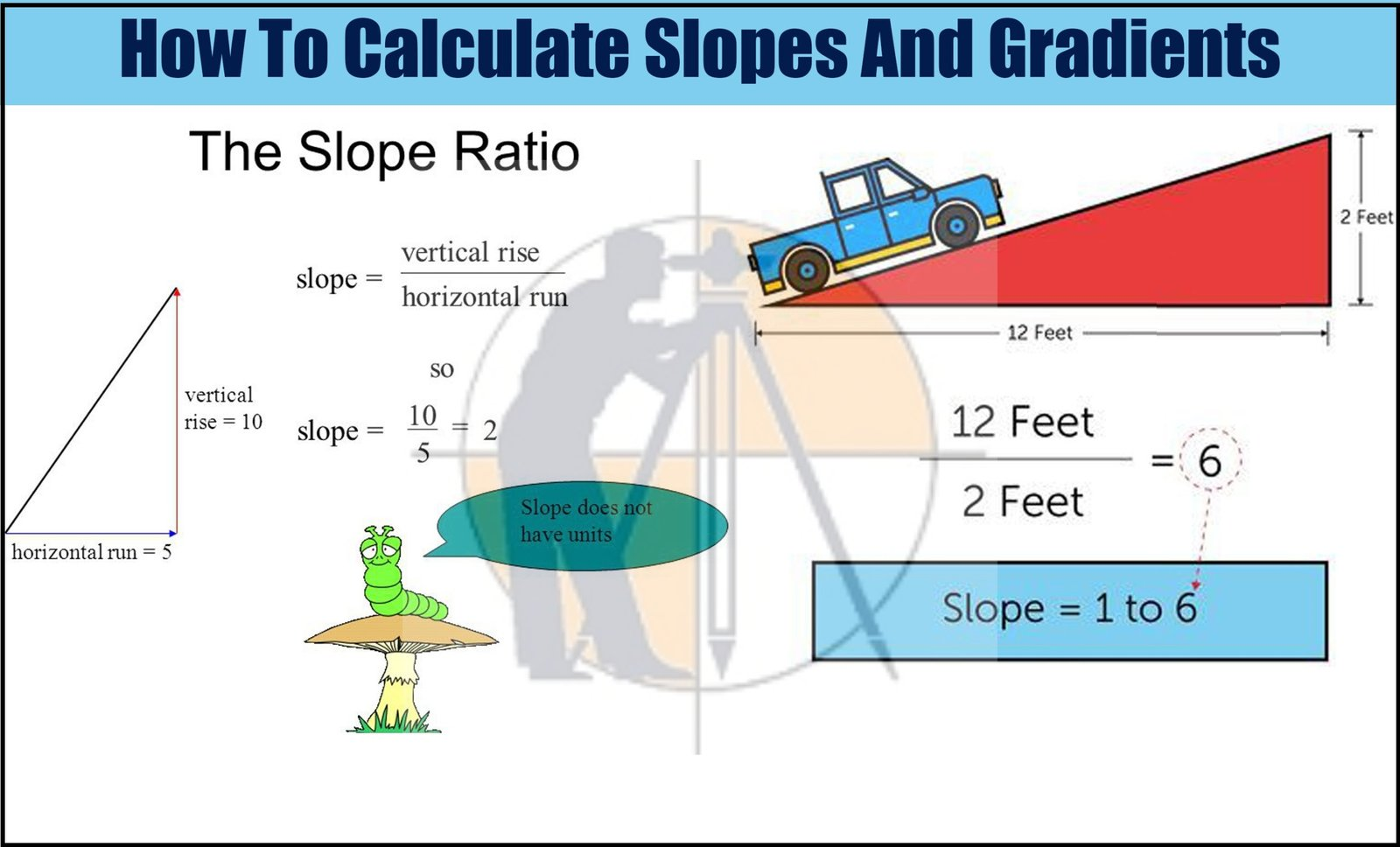Breaking News
Home / Land Surveying / How to calculate The gradient, run and rise in civil construction

# How to calculate The gradient, run and rise in civil construction

### How to Calculate Gradient, Run and Rise in Civil Construction.

In this Article i will calculate the slope, run & rise in the three different drawings as shown below.#### 1 – Slope calculation.Given Data.

Run = 15m.

Rise = 0.5m.

Slope = [run ÷ rise]

= [15m. ÷ 0.5m].

= 30

5. ### Super-elevation And Design Formula | Advantages | Highway Engineering

So, we should provide the gradient or slope of 1: 30

#### 2 – Run calculation.Given Data.

Slope = 1: 100

Rise = 0.5m

Run

= [ slope x rise ]

= [ 100 x 0.5 ]

= Fifty m.

So, we have to provide a ( Fifty m ) run for every 0.5m rise.

#### 3 – Rise calculation.Given Data.

Run = Eleven m.

Rise

= [ run ÷ slope ]

= [ 12m. ÷ 30 ]

= 0.4-m. or 400-mm.

4. ### Basic Thumb Rules For Civil Engineers

So, for a 12m run, u have to provide the 0.4m. or 400mm. rise.

#### 4 – Rise per meter calculation.When we make roads, we cannot provide the rise for the total length as the work should be done in the different phases. In such cases, we have to calculate rise per meter run.

Rise

= [ run ÷ slope]

= [ 200m. ÷ 100 ]

= Two m

Here,

For 200m run,  rise = Two m

For  One m. run,    rise =?

By cross multiplication,

[One m. × Two m.] = [rise/m. × 200]

Rise/m. = [( One m. × Two m. ) ÷200 ]

0.01m. or Ten mm.

If you want to provide a gradient for the Twenty m. run of the above-given road,

The formula is

= [ rise/m. × run length ]

=  [ 0.01m per m. × Twenty m. ]

= 0.2m. or 200mm.

## . Land Surveying & Architects

### THANKS.